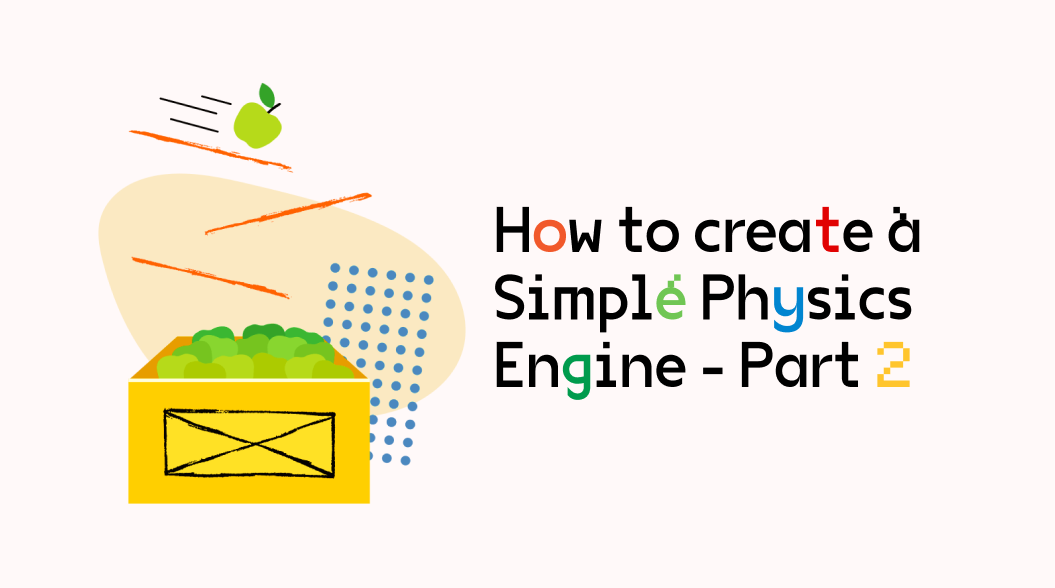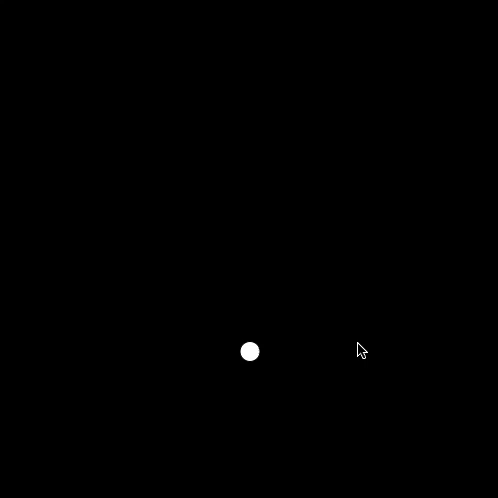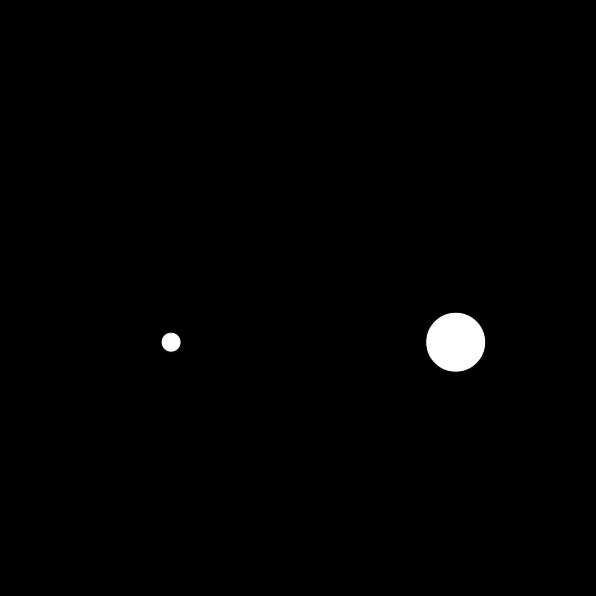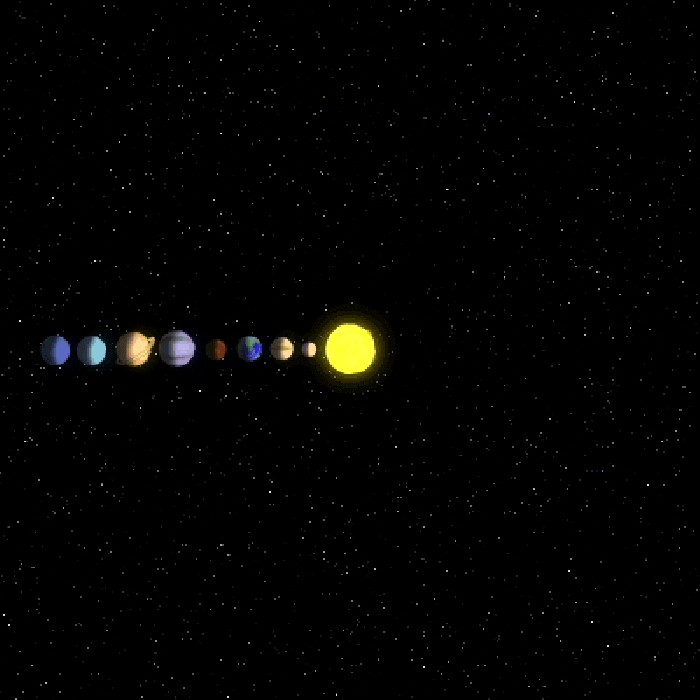# How to create a Simple Physics Engine - Part 2

In this second part of creating a Simple Physics Engine, we dive into some advanced topics like Acceleration, Velocity and Force. Read on to learn moreKarthik KamalakannanNow that we have understood the littlest amount of physics in code, we will go further to make our engine more physics realistic. In the last tutorial, I promised at the end to look at Mass, Force, and Acceleration. Indeed we will be concentrating on these here.

Since we know that F=ma from Smart Boy Newton, we will work with Acceleration first before going to Force.

#### What is Acceleration? What does acceleration do to you? What do you need for something to accelerate?

"Acceleration is the rate of change of velocity of an object with respect to time."

Rate of change of velocity => Acceleration

#### What is the velocity?

"Velocity is the rate of change of position of an object with respect to time"

Rate of change of position => Velocity

We already wrote the change of position in the last code example. Now we will see how to implement acceleration.

Acceleration:

Acceleration can be of 2 types

1. Constant
2. Random

Constant Acceleration:

When the rate of change of velocity is in the same direction, then the acceleration is constant. Okay hold on, too many technical terms, JAGGANAAAATH - moves with constant acceleration.Random Acceleration:

When the rate of change of velocity is … Hold on when your object does not move in a straight line like JAGGANAAAAT … you get random acceleration.

Let's take a look at the code for constant acceleration first

sketch.js

``````let ball;

function setup() {
createCanvas(500, 500);
ball:new Ball();
}

function draw() {
background(0);
ball.move();
ball.bounce();
ball.show();
}``````

We will just create a ball object and write 3 functions in the ball class to move, bounce and show the object.

Ball.js

``````class Ball {
constructor(){
this.location:createVector(width/2,height/2);
this.velocity:createVector(0,0);
this.acceleration:createVector(0,0.5);
}

move(){
}

bounce(){
if(this.location.x<10){
this.location.x:10;
this.velocity.x:-this.velocity.x;
}
else if(this.location.x>width-10){
this.location.x:width-10;
this.velocity.x:-this.velocity.x;
}
if(this.location.y<10){
this.location.y:10;
this.velocity.y:-this.velocity.y;
}
else if(this.location.y>height-10){
this.location.y:height-10;
this.velocity.y:-this.velocity.y;
}
}

show(){
fill(255);
ellipse(this.location.x , this.location.y , 20 ,20);
}
}``````

That's it just 36 lines of code.

We will just initialize the object to start at the center of the screen, with a velocity of 0 and an acceleration of 0.5 on the positive y-axis ( meaning downwards ).

Next, we will move the ball with the move() function. We already know that we can move the ball with a linear motion by changing the velocity vector. But now we have an additional vector called the.

So as per the definition,

Velocity => change in position ( sum the location vector to velocity vector )

Acceleration => change in velocity ( sum the velocity vector to the acceleration vector )

Technically you are done, the bounce() function exists to only check if the object hits the boundaries or not ( It is pretty self-explanatory).

Finally, the show() function just shows the object at its current location ( sum of everything )

And here is the output:Now let's talk about Random Acceleration

We will take the same example

``````let ball;

function setup() {
createCanvas(500, 500);
ball:new Ball();
}

function draw() {
background(0);
ball.move();
ball.bounce();
ball.show();
}``````

In the Ball.js class, we will just change something and add new lines

``````class Ball {
constructor(){
this.location:createVector(width/2,height/2);
this.velocity:createVector(0,0);
this.limit:10;
}

move(){
this.acceleration:p5.Vector.random2D();
this.velocity.limit(this.limit);
}

bounce(){
if(this.location.x<10 || this.location.x>width-10){
this.velocity.x:this.velocity.x * -1;
}

if(this.location.y<10 || this.location.y>height-10){
this.velocity.y:this.velocity.y * -1;
}
}

show(){
fill(255);
ellipse(this.location.x , this.location.y , 20 ,20);
}
}``````

Now, p5 offers a vector which is random and 2D (meaning only x and y contained in the vector) so that you don't have to write a random function (of course you could try to write it yourself).

In our constructor class, we don't define our initial acceleration because we know that we want our acceleration to be random.

Instead, we have a limit value which is a property of p5 vectors that limits all its magnitudes to the limit value. We have just added that so that our object doesn't get out of control by accelerating too much.

We definitely don't want this to happen

So we will limit the velocity since it is the one which will eventually determine the position of the object.

We will now add this random acceleration to our ball object and see what it looks like.

And here is the output:What is a force? What causes a force to be exerted? What do you need to add force?Force is a push or pull on an object with mass that causes it to change velocity (to accelerate).

So we have the velocity, we have the acceleration let's code force. Hold on, where is massthen??

Yes, mass is a very important term which influences the force applied or exerted. But if we assume that our code objects are all of the same mass which is "1" so that mass is canceled out of the equation, it becomes F:a

What we just created (the constant acceleration is the same as when the mass is 1)

Now we will start considering mass. There are many types of forces out there like

• Applied Force.
• Gravitational Force.
• Normal Force.
• Frictional Force.
• Air Resistance Force.
• Tension Force.
• Spring Force.

We will first see how to apply a basic force, frictional force and gravitational force.

Basic force with mass:

For this example we will take 2 objects (1 with smaller mass and 1 with a larger mass) and apply 2 forces (the force of gravity and the force of wind).

Sketch.js

``````let ball:\[\];

function setup() {
createCanvas(600, 600);
for(var i:0; i< 2 ; i++){
ball\[i\]:new Ball(((i/2)+0.25)*width,height/2,i);
}
}

function draw() {
background(0);
for(var i:0; i<ball.length; i++){
//apply the force of gravity
let gravity:createVector(0,0.2);
gravity.mult(ball\[i\].mass);
ball\[i\].applyForce(gravity);

let wind:createVector(0.1,0);
ball\[i\].applyForce(wind);
ball\[i\].move();
ball\[i\].bounce();
ball\[i\].show();
}
}``````

We will create 2 balls and apply the force of gravity and wind.

For gravity, we must multiply the mass of the object because Smart Boy Newton's law of universal gravitation states that every particle attracts every other particle in the universe with a force which is directly proportional to the product of their masses and inversely proportional to the square of the distance between their centers.

So masses need to be considered when applying the force of gravity.

Ball.js

``````class Ball {
constructor(x,y,m){
this.location:createVector(x,y);
this.velocity:createVector(0,0);
this.acceleration:createVector(0,0);
this.mass:(m+0.5)*2;
}

applyForce(force){
this.f:force.div(this.mass)
}

move(){
this.acceleration.mult(0);
}

bounce(){
if(this.location.x<(this.mass_20)/2){
this.location.x:(this.mass_20)/2;
this.velocity.x:-this.velocity.x;
}
else if(this.location.x>width-(this.mass_20)/2){
this.location.x:width-(this.mass_20)/2;
this.velocity.x:-this.velocity.x;
}
if(this.location.y<(this.mass_20)/2){
this.location.y:(this.mass_20)/2;
this.velocity.y:-this.velocity.y;
}
else if(this.location.y>height-(this.mass_20)/2){
this.location.y:height-(this.mass_20)/2;
this.velocity.y:-this.velocity.y;
}
}

show(){
fill(255);
ellipse(this.location.x , this.location.y , this.mass_20 , this.mass_20 );
}
}``````

This snippet is exactly the same as the one before, except the fact that we have to just include a generic force method.

Now we have the force being passed, and also the mass of the object. From this, we calculate the acceleration.

F:ma

So, a:F/m

Now we get the accelerating force. Just add it to the velocity.

And voila, we have:Frictional force:

Friction is the force resisting the relative motion of solid surfaces, fluid layers, and material elements sliding against each other.Friction is easy to implement because we know what to do. Since we are working with vectors, we need to normalize the velocity and multiply it by the coefficient of viscosity of the other object.

Simple, make the length of the velocity 1 and multiply by the thickness of the other material.

In this example, we will use a Ball and a Liquid, and as soon as the ball is inside the liquid, it gets hit by friction.

sketch.js

``````let ball;
let liquid;

function setup() {
createCanvas(600, 600);
ball:new Ball(width/2,0);
liquid:new Liquid(0, height/2);
}

function draw() {
background(0);
//apply the force of gravity
let gravity:createVector(0,0.2);
gravity.mult(ball.mass);
if(ball.inside(liquid)) {
let friction:ball.velocity.copy();
friction.normalize();
let c:-0.5;
friction.mult(c);
ball.applyForce(friction)
}
ball.applyForce(gravity);
ball.move();
ball.bounce();
liquid.show();
ball.show();
}``````

Same as the gravity code, but now liquid is another object and ball object now has to check if the ball location is inside the liquid.

If the ball object's location is inside the liquid, then the ball experiences frictional force + gravity.

Ball.js

``````class Ball {
constructor(x,y){
this.location:createVector(x,y);
this.velocity:createVector(0,0);
this.acceleration:createVector(0,0);
this.mass:4;
}

inside (liquid) {
if(this.location.y >= liquid.location.y) {
return true;
}
else {
return false;
}
}

applyForce(force){
this.f:force.div(this.mass)
}

move(){
this.acceleration.mult(0);
}

bounce(){
if(this.location.x<(this.mass*20)/2){
this.location.x:(this.mass*20)/2;
this.velocity.x:-this.velocity.x;
}
else if(this.location.x>width-(this.mass*20)/2){
this.location.x:width-(this.mass*20)/2;
this.velocity.x:-this.velocity.x;
}
if(this.location.y<(this.mass*20)/2){
this.location.y:(this.mass*20)/2;
this.velocity.y:-this.velocity.y;
}
else if(this.location.y>height-(this.mass*20)/2){
this.location.y:height-(this.mass*20)/2;
this.velocity.y:-this.velocity.y;
}
}

show(){
fill(255);
ellipse(this.location.x , this.location.y , this.mass*20 , this.mass*20   );
}
}``````

The inside() function takes the liquid object as its parameter and checks if the current ball object's y location is greater than or equal to the liquid's y location. If the ball's y location is greater than the y location of the liquid object, then the ball is inside the liquid and returns true.

And then in sketch, if the inside() function returns true, ball object gets the friction force added as

let friction:ball.velocity.copy(); friction.normalize(); let c:-0.5; friction.mult(c); ball.applyForce(friction)

• We will initially make a copy of the ball object's velocity.
• Normalize the velocity vector ( meaning make its magnitude to be equal to 1 or simply make the vector length be 1 ).
• Get the value of 'c', c is the coefficient of viscosity.
• Now multiply the normalized vector by the coefficient of viscosity.
• Finally, apply that force to the object.

And your final output is :And there you have it, FORCE.

With the principles and the simple logic used here, you can experiment with them in a large number of ways to create your own perfectly toned engine or if you wanna go crazy, and create a wacky and funny engine. In our next briefing, we will discuss

Planetary orbitsAnd in upcoming discussions, we will go through

• Fluid physics
• Rigid Body physics
• Soft Body Physics

Last updated: November 21st, 2023 at 6:56:16 PM GMT+0

Subscribe

Get notified about new updates on Product Management, Building SaaS, and more.Company

Ventures

Resources